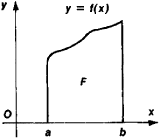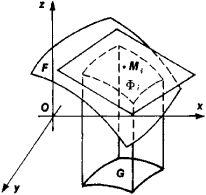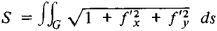# area

(redirected from area germinativa)
Also found in: Dictionary, Thesaurus, Medical, Legal, Financial.

## area

area, measure of the size of a surface region, usually expressed in units that are the square of linear units, e.g., square feet or square meters. In elementary geometry, formulas for the areas of the simple plane figures and the surface areas of simple solids are derived from the linear dimensions of these figures. Examples are given in the table entitled Formulas for Various Areas. The areas of irregular figures, plane or solid, can be computed or closely approximated by the use of integral calculus.

The following article is from The Great Soviet Encyclopedia (1979). It might be outdated or ideologically biased.

## Area

a fundamental quantity associated with geometric figures. In the simplest case it is measured by the number of unit squares—that is, squares with sides of unit length—that fill a plane figure.

The calculation of areas was already in antiquity one of the most important problems of practical geometry because of its role in the division of parcels of land. Several centuries before the Common Era, Greek geometers possessed exact rules for the calculation of areas. In Euclid’s Elements these rules were stated in the form of theorems. The areas of polygons were determined by the same methods of decomposition and complementation of figures used today as teaching devices in schools. The areas of figures with curvilinear boundaries were calculated by the method of exhaustion, which, in a certain sense, involved passage to a limit.

The theory of areas of plane figures bounded by simple curves —that is, curves that do not intersect themselves—can be constructed as follows. Let us consider every polygon that can be inscribed in and every polygon that can be circumscribed about a figure F. It should be noted, incidentally, that the area of a polygon can be calculated by calculating the area of an equivalent square obtained by making suitable rectilinear cuts and rearranging the parts obtained. Let {Si} be the number set of areas of the polygons inscribed in the figure, and let {Sd} be the number set of areas of the polygons circumscribed about the figure. [Si] is bounded from above by the area of any circumscribed polygon, and {Sd} is bounded from below by, for example, zero. The least upper bound S̄ of {Si} is called the linear area of F, and the greatest lower bound of {Sd} is the outer area of F. If the inner area of the figure coincides with its outer area, the number S = S̱ – S̄ is called its area, and the figure is said to be squarable (Jordan measurable). A necessary and sufficient condition for a plane figure to be squarable is that for any positive number ∊ there exist a polygon circumscribed about the figure and a polygon inscribed in the figure such that the difference Sd – Si of their areas is less than ∊.Figure 1

Analytically, the area of a plane figure can be calculated by means of integrals. Suppose F is a curvilinear trapezoid (Figure 1) bounded by the graph of the continuous and nonnegative function f(x) defined on the interval [a, b], by segments of the lines x = a and x = b, and by the segment of the x-axis between the points (a, 0) and (b, 0). The area of the figure can be expressed by the integralThe area of a figure bounded by a closed curve that intersects a line parallel to the y-axis at most twice can be calculated as the difference between the areas of two figures similar to a curvilinear trapezoid. The area of the figure can be expressed in the form of the double integral

S = ∬ dx dy

where the integration extends over the part of the plane occupied by the figure.

The theory of areas of figures on a curved surface can be defined in the following way. Suppose F is a simply connected figure on a smooth surface bounded by a piecewise smooth curve. Let F be partitioned by piecewise smooth curves into a finiteFigure 2

number of parts Φi, each of which has a well-defined projection in the plane tangent to the surface at the point Mi of Φi (Figure 2). Associate to each partition of F the sum of the areas of the projections of all its elements. If for every sequence of partitions of F with diameters approaching zero the corresponding sequence of sums tends to a limit that is independent of the choice of the points Mi, then this limit is defined to be the area of F If F has area, then it is said to be squarable (Jordan measurable). Piecewise smooth, bounded, closed, two-sided surfaces are squarable. The area of a surface is the sum of the areas of its components.

Analytically, the area of a figure F on a surface defined by the equation z = f(x, y), where f is single-valued and has continuous partial derivatives, is given byHere, G is the closed region that is the projection of F onto the xy-plane, and ds is the element of area of the plane.

### REFERENCES

Fikhtengol’ts, G. M. Kurs differential’nogo i integral’nogo ischisleniia, 7th ed., vol. 2. Moscow, 1969.
Kudriavtsev, L. D. Matematicheskii analiz, vols. 1-2. Moscow, 1970.
Il’in, V. A., and E. G. Pozniak. Osnovy matematicheskogo analiza, 3rd ed., parts 1-2. Moscow, 1971-73.

## area

[′er·ē·ə]
(computer science)
A section of a computer memory assigned by a computer program or by the hardware to hold data of a particular type.
(mathematics)
A measure of the size of a two-dimensional surface, or of a region on such a surface.
McGraw-Hill Dictionary of Scientific & Technical Terms, 6E, Copyright © 2003 by The McGraw-Hill Companies, Inc.

## area

1. Measurement of surface within specified boundaries.
2. Space either within or outside a structure or location, designated for a specific purpose, as recreation and/or parking area.
3. An uninterrupted interior space.
4. An areaway.
5. The cross-sectional area of steel reinforcement.
McGraw-Hill Dictionary of Architecture and Construction. Copyright © 2003 by McGraw-Hill Companies, Inc.

## area

1.
a. the extent of a two-dimensional surface enclosed within a specified boundary or geometric figure
b. the two-dimensional extent of the surface of a solid, or of some part thereof, esp one bounded by a closed curve
2. Anatomy any of the various regions of the cerebral cortex
3. Computing any part of a computer memory assigned to store data of a specified type
Collins Discovery Encyclopedia, 1st edition © HarperCollins Publishers 2005
Site: Follow: Share:
Open / Close